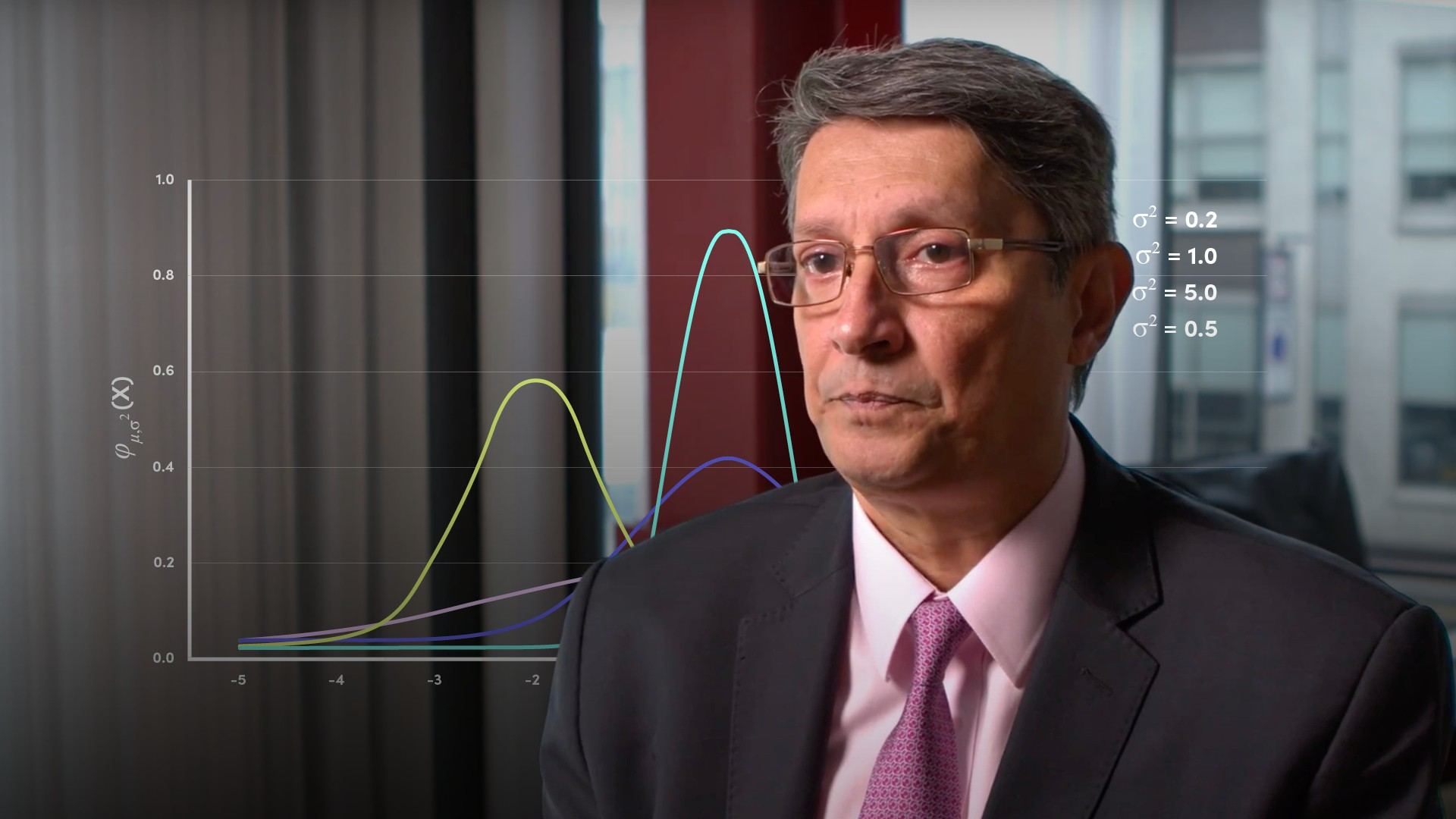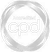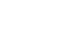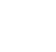# Normal Probability Distributions### Abdulla Javeri

Abdulla explains the normal distribution, which is one of the most widely used of all probability distributions. He discusses its basic characteristics and how to determine probability based on the distance from the average.

Financial MathsAbdulla explains the normal distribution, which is one of the most widely used of all probability distributions. He discusses its basic characteristics and how to determine probability based on the distance from the average.

Speak to an expert

Speak to an expert today to access this and all of the content on our platform.### Normal Probability Distributions

4 mins 57 secs

Overview

The normal distribution is a popular technique in statistics that shows the probability of different outcomes in an experiment. Terms such as mean and standard deviation are crucial in being able to interpret the curve.

Key learning objectives:

• Define and understand what the normal distribution is

• Identify the relationship between mean, standard deviation and the probability of a given outcome

## Speak to an expert

Speak to an expert today to access this and all of the content on our platform.

Summary

#### What is a normal distribution?

The normal distribution is a representation of the probabilities of outcomes. The curve is symmetrical around a central point, the mean. However, just because the curve is symmetrical, or bell shaped, it doesn’t necessarily mean that it’s normally distributed. The term bell shaped seems to have been adopted to actually mean a normal distribution, so we’ll stay consistent with that. On the x axis, the limits are minus infinity and plus infinity, and the curve is asymptotic, which means the curve never touches or crosses the x axis. The x axis represents the outcome of experiments, in other words, numbers or percentages. And the y axis represents the probability of any given outcome.Just by looking at it we can draw some conclusions.

• Firstly, the probability of a particular outcome is related to the distance from the mean
• Secondly, the probability of a particular outcome is related to the standard deviation

#### How is the shape of the curve related to the standard deviation?

We can draw a probability distribution for any level of standard deviation which in turn means that there are an infinite number of normally distributed bell-shaped curves. Some of which are shown in the graphic. The shape of the curve is dependent on the standard deviation. It could be high and narrow if the standard deviation is low, or low and broad if the standard deviation is high.

#### How are the mean and standard deviation related to determining the probability of an outcome?

The units on the x axis are likely to be numbers, prices, percentages, or returns. In a normal distribution the probability of finding a level, a certain distance above the mean, is the same as the probability of finding a level which is the same distance below the mean. For example, if you have a mean of 100, there’s an equal probability of a number being 110 or ten percent above the mean as it is of it being 90 or ten percent below the mean. The y axis shows the probability of each number given a mean and standard deviation. To reiterate, the actual probability associated with each outcome will follow the Gaussian function and be dependent on the distance from the mean and the standard deviation.

A characteristic of the normal distribution is that the area under the curve, namely the probability of the outcome being between -1 and plus 1 standard deviations is always approximately 68%, irrespective of the mean and standard deviation.

## Speak to an expert

Speak to an expert today to access this and all of the content on our platform.### Abdulla Javeri

Abdulla’s career in the financial markets started in 1990 when he entered the trading floor of the London International Financial Futures Exchange, LIFFE, and qualified as a pit trader in equity and equity index options. In 1996, Abdulla became a trainer for regulatory qualifications and then for non-exam courses, primarily covering all major financial products.

There are no available videos from "Abdulla Javeri"

### Accredited by